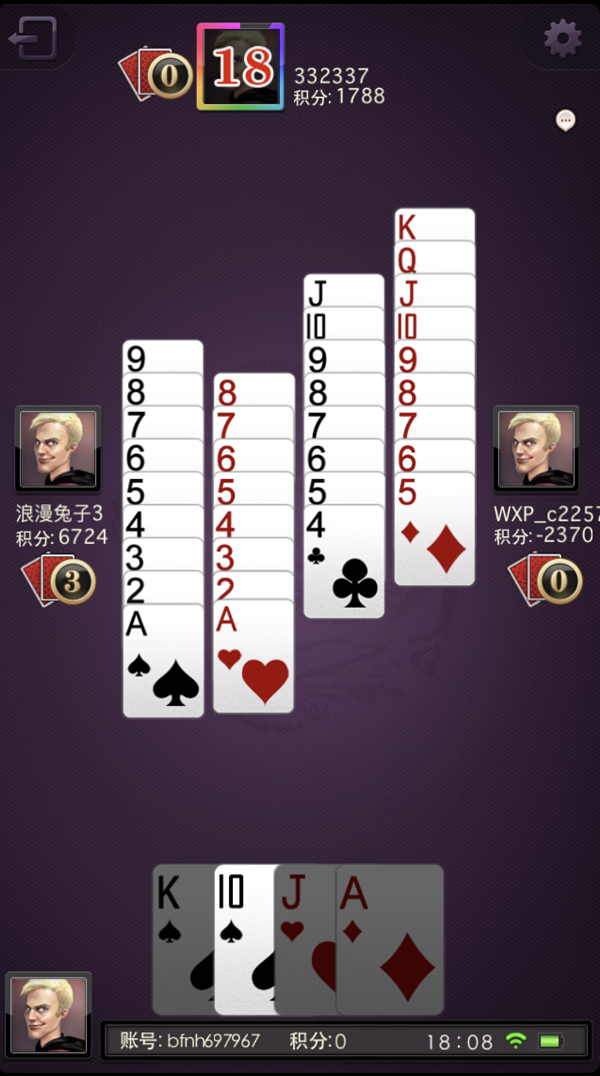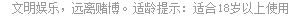# 憋七规则大揭秘A5分；B8分；C1分；D12分。

A的得分=(B扣牌分一A扣牌分)+( C扣牌分一A扣牌分)+(D扣牌分一A扣牌分)

B的得分=(A扣牌分一B扣牌分)+( C扣牌分一B扣牌分)+(D扣牌分一B扣牌分)

C的得分=(A扣牌分一C扣牌分)+(B扣牌分一C扣牌分)+(D扣牌分一C扣牌分)

D的得分：(A扣牌分一D扣牌分)+(B扣牌分一D扣牌分)+(C扣牌分一D扣牌分)

A(85)+(15)+(125)=34+7=6分。

B(58)+(18)+(128)=37+4=6分。

C(51)+(81)+(121)=4+7+11=22分。

D(512)+(812)+(112)=7411=22分。

当有一位玩家“走通”时，其他三位玩家的扣牌分都要加倍；当发生“双通”时，另两位玩家的扣牌分都要乘以4；当发生“三通”时，未通的一位玩家扣牌分要乘以8 每出现一张“独头七”，未通的每位玩家的扣牌分要乘以8

• [07-05]
• [12-06]
• [10-27]
• [06-25]
• [02-02]
• [01-26]
• [01-26]
• [01-26]
• [01-26]
• [01-26]

4007202233

## 官方QQ群

• 茶苑捕鱼：479257360    千变双扣：795175162
• 百变双扣：795642469    四副升级：795182559